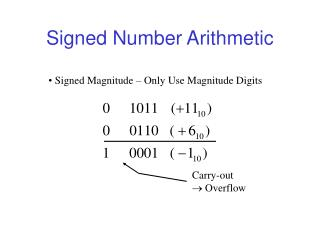Download PresentationSigned Number Arithmetic

Signed Number Arithmetic - PowerPoint PPT Presentation

Signed Number Arithmetic. Signed Magnitude – Only Use Magnitude Digits. Carry-out  Overflow. Radix Complement Arithmetic. Radix Complement; In this case 2’s Complement. Carry-out Does NOT Mean Overflow. 2’s Complement Overflow.I am the owner, or an agent authorized to act on behalf of the owner, of the copyrighted work described.
Download PresentationSigned Number Arithmetic

Download Policy: Content on the Website is provided to you AS IS for your information and personal use and may not be sold / licensed / shared on other websites without getting consent from its author.While downloading, if for some reason you are not able to download a presentation, the publisher may have deleted the file from their server.

- - - - - - - - - - - - - - - - - - - - - - - - - - E N D - - - - - - - - - - - - - - - - - - - - - - - - - -
Presentation Transcript
1. Signed Number Arithmetic • Signed Magnitude – Only Use Magnitude Digits Carry-out  Overflow

2. Radix Complement Arithmetic • Radix Complement; In this case 2’s Complement Carry-out Does NOT Mean Overflow

3. 2’s Complement Overflow • If X, Y have opposite signs overflow never occurs whether carry-out exists or not No Carry-out Carry-out • If X, Y have same sign and result sign differs, overflow occurs No Carry-out,Overflow Carry-out, Overflow

4. 1’s Complement Overflow • One’s complement – carry-out indicates a correction is needed • If X>Y, then answer should be X-Y however; registercontains X-Y-ulp since 2n is carry-out bit, therefore must“correct” by adding 1 ulp

5. Example of 1’s Complement Overflow NeedCorrectionSinceOverflow So-called “end-around” carry

6. 1’s Complement Error • One’s Complement Can Be Better Since Easier to Form Complement • Carry-out in One’s Complement Indicates a Correction is Needed Definition • if X>Y, then answer should be X-Y however register contains X-Y-ulp since 2n is carry-out bit, therefore mustcorrect by adding 1 ulp to answer

7. “End-Around” Carry Design Carry-out • This is “end-around” carry – always add carry-out to LSD

8. Other Number Systems • Binary Number Systems are Most Common • In terms of building “fast” systems, we should consider: • Negative Radix • Signed Digit • Log (logarithm) • Signed Log • Complex Radix • Mixed Radix • Residue Number Systems

9. Negative Radix Fixed Position Systems Nega-decimal example:

10. Nega-Decimal Number System Largest Positive Value, Xmax: Smallest Value, Xmin: Finite Register Length, n=3 digits: Asymmetric System!!!: 10 times more positive than negative values represented

11. Nega-Decimal Number System Finite Register Length, n=4 digits: Now more Negative Values than Positive Nega-decimal System Characteristics: • Arithmetic Operations Same Regardless of Sign of Number • No Signed Digit/Complement Representation Needed • Sign of X Determined by Position of First Non-zero Digit

12. Nega-Binary Number System Negative Radix: Example How is this Addition Operation Performed?????

13. Nega-Binary Number System wi Values (5)10 (-3)10 (1+1=4-2)10 (0+0=0)10 (4+4=16-8)10 (0-8=-8)10 (5-3=2)-10 Carry-out

14. Nega-Binary Adder Design • Individual Adder Cells Produce Two Carry-out Bits • Design a Circuit at Gate Level for a 4-Digit Nega-Binary Adder • Hint: Cout Functions Should Look Familiar!